# Transform | Window M#s

This command is used to multiply all (or selected) M#s by various windowing functions. When it is executed, the following window is opened,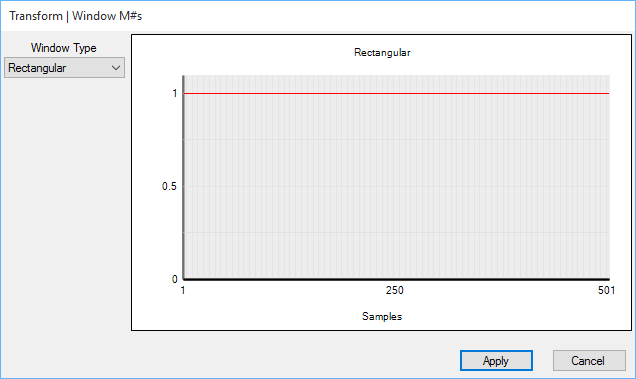Window M#s Dialog Box.

## Notch Window

The Notch window is useful for removing (zeroing) unwanted samples of data. It uses a Cosine function to transition the data smoothly to zero inside the cursor band.  The Notch window is defined as,

• Notch = 1.0 outside the cursor band.

• Notch = Cos(0) =1.0 to Cos(90) = 0.0 from 0% to 5% of the cursor band.

• Notch = 0.0 from 5% to 95% of the cursor band.

• Notch = Cos(90) = 0.0 to Cos(0) = 1.0 from 95% to 100% of the cursor band.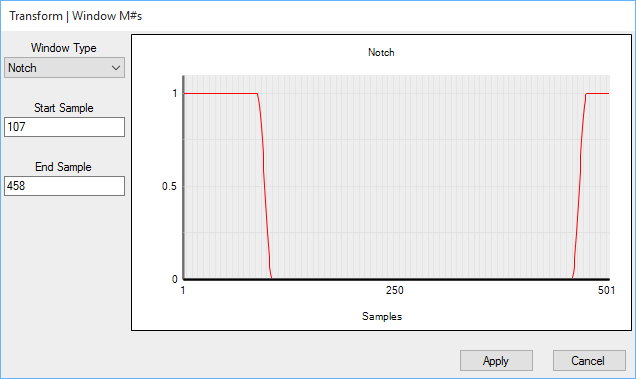Notch Window.

To apply the Notch window,

1. Select the M#s to be windowed.

2. Display the Band cursor, and position the band to enclose the data to be notched (or zeroed).

3. Execute Transform | Window M#s, select the Notch window, and press Apply.

## Band Pass Window

The Band Pass window is useful for preserving certain samples of data and setting the rest to zero.  It uses a Cosine function to transition the data smoothly to zero outside the cursor band.  The Band Pass window is defined as,

• Band Pass = 1.0 inside the cursor band.

• Band Pass = Cos(90) = 0.0 to Cos(0) = 1.0 for 5% of the cursor band prior to the lower edge.

• Band Pass = Cos(0) = 1.0 to Cos(90) = 0.0 for 5% of the cursor band following the upper edge.

• Band Pass = 0.0 otherwise.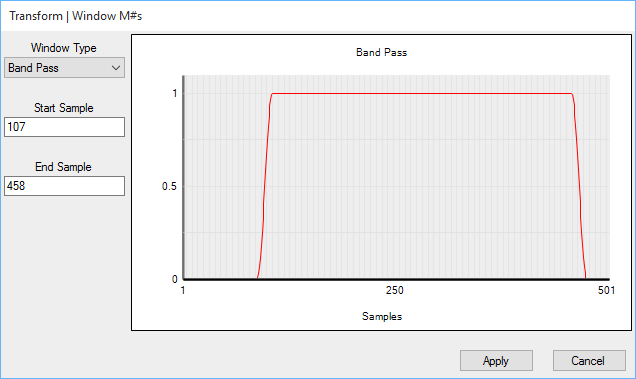Band Pass Window.

To apply the Band Pass window,

1. Select the M#s to be windowed

2. Display the Band cursor, and position the band to enclose the data to be preserved

3. Execute Transform | Window M#s, select the Band Pass window, and press Apply

## Interpolation Window

This window is useful for replacing unwanted data with smooth data in a band of samples.

• Replaces data in the cursor Band with a straight line of data between the data values at the ends of the band.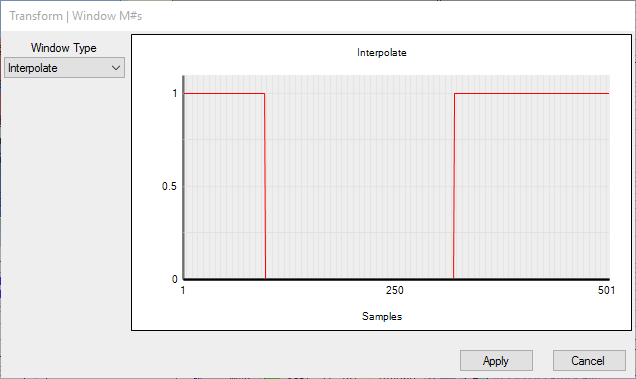Interpolation Window.

## Exponential Window

This window is only applied to time waveforms.  Each time waveform is multiplied by a decreasing (or increasing) exponential curve.

• The beginning value of the exponential window is "1".

• For a decreasing exponential, the ending value of the window should be less than "1" but greater than "0".

• For an increasing exponential window, the ending value should be greater than "1".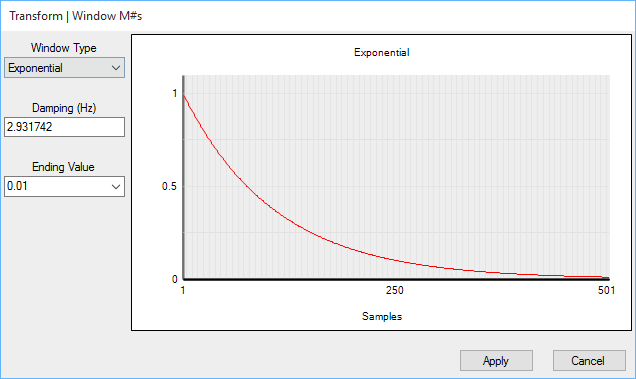Exponential Window.

The following steps are carried out when this window is applied to frequency domain M#s;

1. All (or selected) M#s are transformed to the time domain using the Inverse FFT.

2. The Exponential window is applied.

3. The M#s are transformed back to the frequency domain using the FFT.

## Decreasing Exponential Removes Noise & Leakage

A deceasing exponential has the effect of truncating the noise in each measurement, and also making a signal more completely contained within the window
When a windowed time waveform is transformed to the frequency domain, its spectrum will have less leakage.

## Increasing Exponential Narrows Resonance Peaks

• If an increasing exponential window is applied to impulse response functions (IRFs), it will artificially decrease the amount of damping in all of the modes by a known amount.

• When windowed IRFs are transformed into their corresponding frequency response functions (FRFs), the resonance peaks will be narrower, and closely coupled modes will be more widely separated from one another.

• An increasing exponential window will also amplify the noise in experimental data.

## Modal Damping

Applying an exponential window to measurements that contain responses due to resonant vibration adds a known amount of damping to each of the modes represented in the data.

• A decreasing exponential window adds the same amount of damping to each mode.

• An increasing exponential window subtracts the same amount of damping from each mode.

The amount of damping added to modes by applying an Exponential window is listed in the Transform | Window M#s dialog box, and also in the Window Value column of the M#s spreadsheet.

The amount of modal damping added by the Exponential window is cumulative.

• If the Exponential window is applied several times to the same data, the amount of damping is the sum of the damping amounts added with each application of the window.

## Damping Removal Following Curve Fitting

During modal parameter estimation (or curve fitting), the artificial modal damping added by the Exponential window is not removed  However, the artificial damping is removed from each modal damping estimate when one of the following commands is executed and modal parameters are saved into a Shape Table,

1. Save Shapes button on the Residues, Save Shapes tab is pressed

2. Curve Fit | Shapes | Save Shapes is executed